Home
Hostname: page-component-65dc7cd545-54nbv Total loading time: 0.177 Render date: 2021-07-25T05:51:51.919Z Has data issue: true Feature Flags: { "shouldUseShareProductTool": true, "shouldUseHypothesis": true, "isUnsiloEnabled": true, "metricsAbstractViews": false, "figures": true, "newCiteModal": false, "newCitedByModal": true, "newEcommerce": true, "newUsageEvents": true }Forum of Mathematics, Sigma

#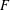$F$-SIGNATURE UNDER BIRATIONAL MORPHISMS

Published online by Cambridge University Press:  17 April 2019

Corresponding

## Abstract

We study$F$-signature under proper birational morphisms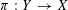$\unicode[STIX]{x1D70B}:Y\rightarrow X$, showing that$F$-signature strictly increases for small morphisms or if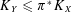$K_{Y}\leqslant \unicode[STIX]{x1D70B}^{\ast }K_{X}$. In certain cases, we can even show that the$F$-signature of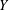$Y$ is at least twice as that of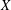$X$. We also provide examples of$F$-signature dropping and Hilbert–Kunz multiplicity increasing under birational maps without these hypotheses.

## MSC classification

Type
Research Article
Information
Creative CommonsThis is an Open Access article, distributed under the terms of the Creative Commons Attribution licence (http://creativecommons.org/licenses/by/4.0/), which permits unrestricted re-use, distribution, and reproduction in any medium, provided the original work is properly cited.

## References

Aberbach, I. M. and Enescu, F., ‘The structure of F-pure rings’, Math. Z. 250(4) (2005), 791806.CrossRefGoogle Scholar
Aberbach, I. M. and Leuschke, G. J., ‘The F-signature and strong F-regularity’, Math. Res. Lett. 10(1) (2003), 5156.CrossRefGoogle Scholar
Bhatt, B., Gabber, O. and Olsson, M., ‘Finiteness of étale fundamental groups by reduction modulo$p$ ’, Preprint, 2017, ArXiv e-prints.Google Scholar
Birkar, C., ‘Existence of flips and minimal models for 3-folds in char p ’, Ann. Sci. Éc. Norm. Supér. (4) 49(1) (2016), 169212.CrossRefGoogle Scholar
Blickle, M. and Schwede, K., ‘ p -1 -linear maps in algebra and geometry’, inCommutative Algebra (Springer, New York, 2013), 123205.CrossRefGoogle Scholar
Blickle, M., Schwede, K. and Tucker, K., ‘ F-signature of pairs and the asymptotic behavior of Frobenius splittings’, Adv. Math. 231(6) (2012), 32323258.CrossRefGoogle Scholar
Bruns, W. and Vetter, U., Determinantal Rings, Lecture Notes in Mathematics, 1327 (Springer, Berlin, 1988).CrossRefGoogle Scholar
Carvajal-Rojas, J., Schwede, K. and Tucker, K., ‘Fundamental groups of F-regular singularities via F-signature’, Ann. Sci. Éc. Norm. Supér. (4) 51(4) (2018), 9931016.CrossRefGoogle Scholar
De Stefani, A., Polstra, T. and Yao, Y., ‘Globalizing$F$ -invariants’, Preprint, 2016, ArXiv e-prints.Google Scholar
Fedder, R., ‘ F-purity and rational singularity’, Trans. Amer. Math. Soc. 278(2) (1983), 461480.Google Scholar
de Fernex, T., Küronya, A. and Lazarsfeld, R., ‘Higher cohomology of divisors on a projective variety’, Math. Ann. 337(2) (2007), 443455.CrossRefGoogle Scholar
Glassbrenner, D., ‘Strongly F-regularity in images of regular rings’, Proc. Amer. Math. Soc. 124(2) (1996), 345353.CrossRefGoogle Scholar
Hara, N. and Yoshida, K.-I., ‘A generalization of tight closure and multiplier ideals’, Trans. Amer. Math. Soc. 355(8) (2003), 31433174 (electronic).CrossRefGoogle Scholar
Huneke, C. and Leuschke, G. J., ‘Two theorems about maximal Cohen–Macaulay modules’, Math. Ann. 324(2) (2002), 391404.CrossRefGoogle Scholar
Huneke, C. and Swanson, I., Integral Closure of Ideals, Rings, and Modules, London Mathematical Society Lecture Note Series, 336 (Cambridge University Press, Cambridge, 2006).Google Scholar
de Jong, A. J., ‘Smoothness, semi-stability and alterations’, Publ. Math. Inst. Hautes Études Sci. 83 (1996), 5193.CrossRefGoogle Scholar
Kollár, J. and Mori, S., Birational Geometry of Algebraic Varieties, Cambridge Tracts in Mathematics, 134 (Cambridge University Press, Cambridge, 1998). With the collaboration of C. H. Clemens and A. Corti, Translated from the 1998 Japanese original.CrossRefGoogle Scholar
Kunz, E., ‘Characterizations of regular local rings for characteristic p ’, Amer. J. Math. 91 (1969), 772784.CrossRefGoogle Scholar
Lazarsfeld, R., ‘Classical setting: line bundles and linear series’, inPositivity in Algebraic Geometry. I, Ergebnisse der Mathematik und ihrer Grenzgebiete. 3. Folge. A Series of Modern Surveys in Mathematics [Results in Mathematics and Related Areas. 3rd Series. A Series of Modern Surveys in Mathematics], 48 (Springer, Berlin, 2004).Google Scholar
Lazarsfeld, R. and Mustaţă, M., ‘Convex bodies associated to linear series’, Ann. Sci. Éc. Norm. Supér. (4) 42(5) (2009), 783835.CrossRefGoogle Scholar
Li, C., Liu, Y. and Xu, C., ‘A guided tour to normalized volume’, Preprint, 2018, ArXiv e-prints.Google Scholar
Liu, Y., ‘The$F$ -volume of singularities in positive characteristic’, 2018, in preparation.Google Scholar
Liu, Y. and Xu, C., ‘$K$ -stability of cubic threefolds’, Preprint, 2017, ArXiv e-prints.Google Scholar
Murayama, T., ‘The gamma construction and asymptotic invariants of line bundles over arbitrary fields’, Preprint, 2018, ArXiv e-prints.Google Scholar
Mustaţă, M., ‘The non-nef locus in positive characteristic’, inA Celebration of Algebraic Geometry, Clay Math. Proc., 18 (American Mathematical Society, Providence, RI, 2013), 535551.Google Scholar
Polstra, T., ‘Uniform bounds in F-finite rings and lower semi-continuity of the F-signature’, Trans. Amer. Math. Soc. 370(5) (2018), 31473169.CrossRefGoogle Scholar
Polstra, T. and Tucker, K., ‘ F-signature and Hilbert–Kunz multiplicity: a combined approach and comparison’, Algebra Number Theory 12(1) (2018), 6197.CrossRefGoogle Scholar
Sannai, A., ‘On dual F-signature’, Int. Math. Res. Not. IMRN 2015(1) (2015), 197211.CrossRefGoogle Scholar
Schwede, K. and Smith, K. E., ‘Globally F-regular and log Fano varieties’, Adv. Math. 224(3) (2010), 863894.CrossRefGoogle Scholar
Smith, K. E. and Van den Bergh, M., ‘Simplicity of rings of differential operators in prime characteristic’, Proc. Lond. Math. Soc. (3) 75(1) (1997), 3262.CrossRefGoogle Scholar
Tucker, K., ‘ F-signature exists’, Invent. Math. 190(3) (2012), 743765.CrossRefGoogle Scholar
Xu, C., ‘Finiteness of algebraic fundamental groups’, Compos. Math. 150(3) (2014), 409414.CrossRefGoogle Scholar
Yao, Y., ‘Observations on the F-signature of local rings of characteristic p ’, J. Algebra 299(1) (2006), 198218.CrossRefGoogle ScholarYou have AccessOpen access
1
Cited by

# Send article to Kindle

Note you can select to send to either the @free.kindle.com or @kindle.com variations. ‘@free.kindle.com’ emails are free but can only be sent to your device when it is connected to wi-fi. ‘@kindle.com’ emails can be delivered even when you are not connected to wi-fi, but note that service fees apply.

Find out more about the Kindle Personal Document Service.$F$-SIGNATURE UNDER BIRATIONAL MORPHISMS Available formats × # Send article to Dropbox To send this article to your Dropbox account, please select one or more formats and confirm that you agree to abide by our usage policies. If this is the first time you use this feature, you will be asked to authorise Cambridge Core to connect with your <service> account. Find out more about sending content to Dropbox.$F$-SIGNATURE UNDER BIRATIONAL MORPHISMS
Available formats
×

# Send article to Google Drive

To send this article to your Google Drive account, please select one or more formats and confirm that you agree to abide by our usage policies. If this is the first time you use this feature, you will be asked to authorise Cambridge Core to connect with your <service> account. Find out more about sending content to Google Drive.$F\$-SIGNATURE UNDER BIRATIONAL MORPHISMS
Available formats
×
×